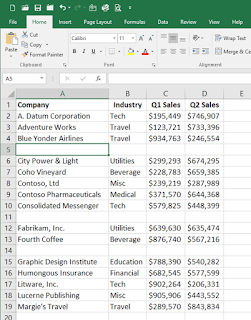## Friday, November 27, 2009

### Add Row Numbers in Excel

There are any number of formulas you can use in column A that will return a row number.

Perhaps the easiest is to use the ROW function, like this:

=ROW( )
This formula returns the row number of the cell in which the formula appears.

If you want to offset the row number returned (for instance, if you have some headers in rows 1 and 2 and you want cell A3 to return a row value of "1", then you can modify the formula to reflect the desired adjustment:
=ROW( )-2

Of course, the ROW function isn't the only formula that will perform this function. Look for more Go Ask Debbie Tips on using Excel formulas and functions directly at Go Ask Debbie.